# Maths - HoTT Equality Examples

On the HoTT page we looked at Voevodsky's 'univalence axiom' (A=B)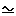(AB). (identity is equivalent to equivalence).

On this page we try to investigate examples of this equivalence which is like isomorphism.

One way to interpret this might be that isomorphism is like equality in multiple ways.

### Equality of Bool

 For instance, in the Boolean example, if we set: 0 = false 1 = true then the sets are equal.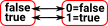Alternatively if we set: 0 = true 1 = false then the sets are equal in a different way.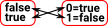There is a higher order morphism (a natural transformation) called 'not' between these 'equalities.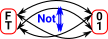Here is the naturality square: This square needs to commute.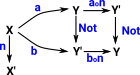### Equality of Finite Set

 For the next example lets go from a 2 element set to a 3 element set. If we have a set with 3 elements there are 6 ways that it can be equal to another such set (the number of permutations of 3 elements = !3 = 6. There is a natural transformation between each of these 6 'equalities' and every other one (I've only shown some of them, in blue, on the diagram. Lets look at equality between two 3-element sets, say, `data primary = R | G | B` and `data secondary C | M | Y`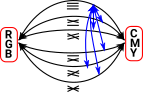Each of these natural transformations has an inverse going in the opposite direction.

We can see how this has the structure of a groupoid. For instance, every natural transformation has an inverse.

More about groupoids on the page here

### Higher Order Equality

 What happens if we have a two element set whose elements are sets? Can we relate the morphisms between the inner sets to morphisms between the outer sets?Iv'e tried to draw a hierachy of naturality squares.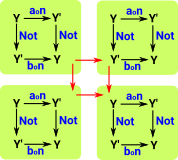Where I can, I have put links to Amazon for books that are relevant to the subject, click on the appropriate country flag to get more details of the book or to buy it from them.Computation and Reasoning - This book is about type theory. Although it is very technical it is aimed at computer scientists, so it has more discussion than a book aimed at pure mathematicians. It is especially useful for the coverage of dependant types.